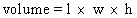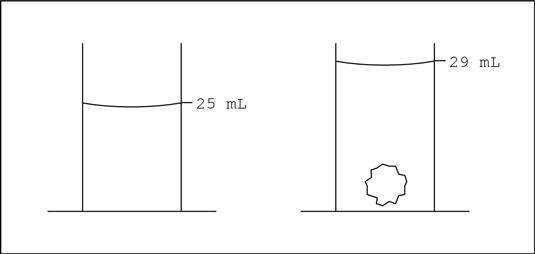##### Chemistry For DummiesWhen chemists study chemical substances, they examine two types of properties: chemical properties and physical properties. Some physical properties are extensive properties, properties that depend on the amount of matter present. Mass and volume are extensive properties.

Intensive properties, however, don’t depend on the amount of matter present. Color is an intensive property. A large chunk of gold, for example, is the same color as a small chunk of gold. The mass and volume of these two chunks are different (extensive properties), but the color is the same.

Following are descriptions of the two types of properties:

• Chemical properties: These properties enable a substance to change into a brand-new substance, and they describe how a substance reacts with other substances. Does a substance change into something completely new when water is added — like sodium metal changes to sodium hydroxide? Does it burn in air?

• Physical properties: These properties describe the physical characteristics of a substance. The mass, volume, and color of a substance are physical properties, and so is its ability to conduct electricity.

## What is density?

Density is one of the most useful intensive properties of a substance, enabling chemists to more easily identify substances. For example, knowing the differences between the density of quartz and diamond allows a jeweler to check out that engagement ring quickly and easily.

Density (d) is the ratio of the mass (m) to volume (v) of a substance. Mathematically, it looks like this:

d = m/v

Usually, mass is described in grams (g) and volume in milliliters (mL), so density is g/mL.

Because the volumes of liquids vary somewhat with temperature, chemists also usually specify the temperature at which a density measurement is made. Most reference books report densities at 20°C, because it’s close to room temperature and easy to measure without a lot of heating or cooling. The density of water at 20°C, for example, is 1g/mL.

Another term you may sometimes hear is specific gravity (sg), which is the ratio of the density of a substance to the density of water at the same temperature. Specific gravity is just another way for you to get around the problem of volumes of liquids varying with the temperature.

In most cases, the density and specific gravity are almost the same, so it’s common to simply use the density.

## Measuring density

Calculating density is pretty straightforward. You measure the mass of an object by using a balance or scale, determine the object’s volume, and then divide the mass by the volume.

Determining the volume of liquids is easy, but solids can be tricky. If the object is a regular solid, like a cube, you can measure its three dimensions and calculate the volume by multiplying the length by the width by the height:If the object is an irregular solid, like a rock, determining the volume is more difficult. With irregular solids, you can measure the volume by using something called the Archimedes Principle, which states that the volume of a solid is equal to the volume of water it displaces.

Say that you want to measure the volume of a small rock in order to determine its density. Follow these steps:

1. Put some water into a graduated cylinder with markings for every mL and read the volume.

The example in the following figure shows 25 mL.

2. Put the rock in the cylinder, making sure that it’s totally submerged, and read the volume again (29 mL in the figure).

The difference in volume (4 mL, in this example) is the volume of the rock.Determining the volume of an irregular solid: The Archimedes Principle.

Anything with a density lower than water will float when put into water, and anything with a density greater than 1 g/mL will sink.

The following table lists the density of some common materials.

Densities of Typical Solids and Liquids in g/mL
Substance Density
Gasoline 0.68
Ice 0.92
Water 1.00
Table Salt 2.16
Iron 7.86